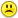+0

# A point is chosen at random on the number line between 0 and 1, and the point is colored green. Then, another point is chosen at random on t

+5
3247
2

A point is chosen at random on the number line between 0 and 1, and the point is colored green. Then, another point is chosen at random on the number line between 0 and 1, and this point is colored purple. What is the probability that the number of the purple point is greater than the number of the green point, but less than twice the number of the green point?

Apr 29, 2015

#1
+16

I think this might be correct, Mellie   .....look at the following pic.....Let the "green" value be an x value lying on segment AC. This segment has the equation y = x. Then, let the "purple" value that is twice a selected x value lie on the segment AE. And the equation of this line is y = 2x. Notice at point E, the x value = .5 and the y value = 1. And at every x value on AC  greater than .5, the  y value on EC associated with this x value is is less than twice that x value.

So, between segments AE and AC, every y value will be greated than, but less than twice, its associated x value. Note that triangle ACD has an area of 1/2. And triangle BEA has an area of 1/4. So, triangle AEC - the area  of interest - has an area of 1 - (1/2) - (1/4)   = 1/4.  And this is the probability that a "purple" value will be greater than, but less than twice,  a "green" value on [0, 1 ].Apr 29, 2015

#1
+16

I think this might be correct, Mellie   .....look at the following pic.....Let the "green" value be an x value lying on segment AC. This segment has the equation y = x. Then, let the "purple" value that is twice a selected x value lie on the segment AE. And the equation of this line is y = 2x. Notice at point E, the x value = .5 and the y value = 1. And at every x value on AC  greater than .5, the  y value on EC associated with this x value is is less than twice that x value.

So, between segments AE and AC, every y value will be greated than, but less than twice, its associated x value. Note that triangle ACD has an area of 1/2. And triangle BEA has an area of 1/4. So, triangle AEC - the area  of interest - has an area of 1 - (1/2) - (1/4)   = 1/4.  And this is the probability that a "purple" value will be greater than, but less than twice,  a "green" value on [0, 1 ].CPhill Apr 29, 2015
#2
+1

Thanks Chris,I wish I had time to do all your great questions MellieApr 30, 2015